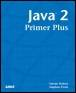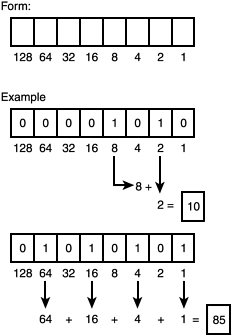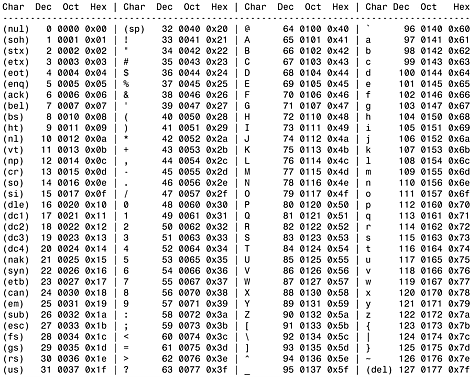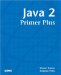# Data TypesJava™ 2 Primer PlusBy Steven Haines, Steve Potts Table of Contents Chapter 2.  Keywords, Data Types, and Variables

A data type is a human-readable tag that represents a specific usage of a computer's memory. When used in a program, it defines the amount of memory that will be used and the valid values that might be placed in that memory.

Java is a strongly typed programming language, meaning that all variables used in a Java program must have a specifically defined data type. A loosely typed programming language, such as JavaScript or Visual Basic, allows the use of the variable to define its type. For example, if a variable is used as a number, then it is a number, if it is used as a string of characters, then it is a string of characters. Because Java is a strongly typed language, we must define how a variable will be used before it can be defined.

#### The Computer/Human Communication Problem

Computers and humans speak two different languages. Humans think of things in terms of objects and define things in terms of numbers, letters, and words. Computers think in terms of 1s and 0s, which represent electrical impulses (1 = impulse, 0 = no impulse).

How can we translate our numbers, characters, and words into the 1s and 0s that computers understand?

Because computers do not have any inherent understanding of the number 5 or the letter B, we must define a representation of these using 1s and 0s, and then write rules that govern how certain operations on these values affect them. We can take the electrical signals, let's call them bits, and group them into groups of 8 bits and call those bytes. So a byte will represent 8 bits, which is comprised of 1s and 0s. How might we represent a number with these 8 bits?

Our alphabet has 26 letters (A through Z), but we are now defining a computer's alphabet as having two "letters": 0 and 1. Because we have only two numbers in our numerical alphabet, we must devise a way to count. In our decimal system we have 10 digits: 0, 1, 2, 3, 4, 5, 6, 7, 8, and 9. In decimal we count as follows: 0 1 2 3 4 5 6 7 8 9, and then we start over with a two digit representation: 10 and proceed 11, 12, 13, and so on. If we count with two digits (binary) we start with 0, 1, and suddenly we are out of numbers. Following the decimal example, we now have our ten: 10, which if we are counting in our familiar decimal notation would be two followed by 11 (or three), and hence we create the sequence: 0 1 10 11 100 101 110 111 1000 (or 1 2 3 4 5 6 7 8).

If we have 8 digits to work with that gives us 256 possible numbers in a single byte. But what about negative numbers? We can designate one bit as representing the sign of the number: Let's say the highest bit (8th in this case) represents the sign (0 is positive and 1 is negative). Now we can represent 128 or 127 negative numbers, zero, and either 127 or 128 positive numbers (we have to count zero as one of our 256 possible numbers). If we were designing Java we would have decided to have 128 negative numbers and 127 positive numbers, thus with one byte, or 8 bits, or 8 electrical signals passing between circuits, we have a mechanism to represent numbers between 128 and +127.

The numbering system we just derived is referred to as binary notation, or base-2, where decimal is base-10. Each bit as we counted up represented a power of 2, see Figure 2.1.

##### Figure 2.1. Binary numbers.As Figure 2.1 shows, we have a definitive way to convert a stream of 1s and 0s into decimal numbers, and vice versa. What if we need larger numbers than 128? We could group bytes together and have multiple bytes represent one single number. For example, if we grouped two bytes together, which is 16 bits, we could designate the high bit to represent the sign, which leaves us with 15 bits to represent our number. 15 bits enables us to now represent the range of numbers 32768 to 32767. We could extrapolate this out further and represent any arbitrary size number we want.

Note that in binary numbers, each successive bit represents double the value that the previous bit represented. That is why that adding an additional 8 bits to our original 128 to 127 range did not simply double the range, but increased it exponentially. This concept of exponential growth is very powerful: Take the entire contents of the Pacific Ocean and dry it up, now start refilling it one drop at a time, doubling the drops each time 1 drop, then 2 drops, then 4 drops, and so on, and by the time you reach the 80th iteration, you have the Pacific Ocean refilled! Powerful stuff!

When we have our bits and bytes, we can define data types, such as numbers and characters, by defining our own interpretation of what these bits mean when associated with a data type. That is all that Java, and every other programming language in the world, has done; only they have involved standards committees to ensure that parts of their interpretation of these bits is global across programming languages. It would be hard to read a text file if Java said that a capital A was one thing and C++ said it was something else.

#### Primitive Data Types

Java defines eight primitive data types, which define the core data that can be represented in the Java programming language. A data type defines the amount of memory that will be used when defining one of the data types and the valid range of values it can represent.

##### Integer Data Types

Integers represent whole numbers (numbers without a fractional part), and Java defines four data types that represent integers:

byte

short

int

long

All integer types are represented as we derived earlier in this chapter: The highest bit represents the sign, whereas the low bits represent the number. The difference between the different integer types is the number of bytes (or bits) grouped together to represent a single number.

Table 2.1 lists the memory usage required for each type and the valid ranges it can represent.

##### Table 2.1. Integer Data Types

Integer Type

Memory Usage

Range of Values

byte

1 byte (8 bits)

128 to +127 ( 27 to 27 1)

short

2 bytes (16 bits)

215 to 215 1int 4 bytes (32 bits) 231 to 231 1

long

8 bytes (64 bits)

263 to 263 1

Choose the integer data type based on the type of data you want to represent. For example, a human age might be represented by a byte or a short (not too many people are older than 32767 years old), whereas the national debt might be better represented by a long.

Here's an example, but don't worry about the Java syntax, we'll talk about that shortly:

` byte b = 50;  short s = 1000;  int i = 500000;  long l = 1000000000000000000000; `
##### Floating-Point Types

Now that we have a type that represents whole numbers, we need a type that represents numbers with a fraction part; this category of numbers is referred to as floating-point types.

Floating-point types are represented by the same bytes that represent integer types, but their interpretation is different. A certain number of bits are used to represent the whole part of the numbers, and a certain number of bits are used to represent the fractional part of the number. The mathematical concept of significant digits is applied with these data types: The number of consecutive numerical entries in the number that can be accurately recorded defines the number of significant digits in the number. For example consider:

` 3.1428571 `

This number has eight significant digits. Whereas

` 120,000,000 `

has only two. 0 is not considered a significant digit unless it is surrounded by two nonzero digits. A common way in mathematics, as well as in computer science, to represent floating-point numbers is to provide an exponential representation of a number. This is defined as listing the significant digits with one digit written before the decimal point, and then defining the power of 10 to multiply by the number to generate its real value. So 120 million could be written as

` 1.2 multiplied by 108 or 1.2E+8 `

From this representation, the number of significant digits should be more apparent.

There are two types of floating-point data types, as shown in Table 2.2.

##### Table 2.2. Floating-Point Data Types

Integer Type

Memory Usage

Range of Values

float

4 bytes (32 bits)

+/ 3.40282347E+38 (6 7 significant digits)

double

8 bytes (64 bits)

+/ 1.7976931346231570E+308 (15 significant digits)

The float data type can represent a large amount of numbers, but only to 6 or 7 digits of precision. If you were representing currency, that would be more than adequate, but if you were calculating the amount of light to apply to a surface in a 3-dimensional graphics application, a double would be more appropriate.

##### Character Type

With numbers out of the way it is time to discuss characters. We use our same set of bits to represent characters, but this time in a tabular lookup form. How much storage is needed to represent characters? In the English language we have 26 uppercase letters, 26 lowercase letters, 10 digits, and an assortment of other characters (+, -, /, *, and so on). But we are very safe in saying that we could represent the English language in 128 characters or less.

But do we arbitrarily give each letter a numerical representation? In the beginning everyone did just that, and it quickly became apparent that machines could not communicate with one another. So, in 1961 Bob Bemer from IBM submitted a numbering scheme that he called the American Standard Code for Information Interchange (ASCII) to the American National Standards Institute (ANSI), a standards body, for approval. In 1968 ASCII was approved as a standard as "ANSI Standardx3.4-1968," and it is the base for our English character set today. Figure 2.2 shows the ASCII table as we know it today.

##### Figure 2.2. ASCII table.We were quite efficient in defining a character set to represent the English language, but how do we represent another language? Do we arbitrarily assign numerical values to their alphabet? How do we differentiate between character sets so that we know what language we are reading? How do we deal with a non-Roman alphabet such as Japanese?

The answer is that we need a much larger set of characters so that we can assign every character in every language a unique value. If we can represent the entire English language in 7 bits (128 characters), how many bits will it take to represent all characters in all languages?

Again, different companies haphazardly created values for different languages until the Unicode Consortium released a standard called Unicode in 1988. It has been through a couple revisions and is currently at version 3.0. This standard uses two bytes (16 bits) to represent a character, which yields more than 1 million different characters; this permits unique characters, no matter the platform, the program, or the language. Following its ASCII heritage, the first 128 characters in the Unicode standard are the ASCII standard.

Java represents all characters in Unicode (again 2 bytes), so all programs that you write will be ready for translation into any other language without requiring you to rewrite the framework of your application.

Characters can be represented in their Unicode form as:

` \uXXXX  Where XXXX is a number in the range of 0000 to FFFF (hexadecimal) `

Or as their English equivalents delimited by single quotes:

` 'a'  'Z'  'n' `

Because characters are delimited by single quotes, Strings (as we will soon learn) are delimited by double quotes, and there are various unprintable characters, Java defines a set of special characters, shown in Table 2.3.

##### Table 2.3. Java Special Characters

Character

Meaning

Unicode Equivalent

\b

Backspace

\u0008

\t

Tab

\u0009

\n

Linefeed

\u000a

\r

Carriage Return

\u000d

\"

Double quote

\u0022

\'

Single quote

\u0027

\\

Backslash

\u005c

Characters in Java are represented by the datatype char.

##### Boolean Data Type

Up to this point we have learned to represent whole numbers, fractional numbers, and characters, so what is left?

The last primitive data type in the Java programming language is the boolean data type. A boolean data type has one of two values: true or false. These are not strings, but keywords in the Java programming language.

Programming languages need to have boolean types so that they can perform specific actions based on predetermined conditions. For example, a program running a traffic light might have a sensor that tells it when people are waiting at the light. If people are waiting at the light (true), the light should prepare itself to change, otherwise it should remain unchanged (false).

 TopJava 2 Primer Plus
ISBN: 0672324156
EAN: 2147483647
Year: 2001
Pages: 332

Similar book on Amazon

flylib.com © 2008-2017.
If you may any questions please contact us: flylib@qtcs.net